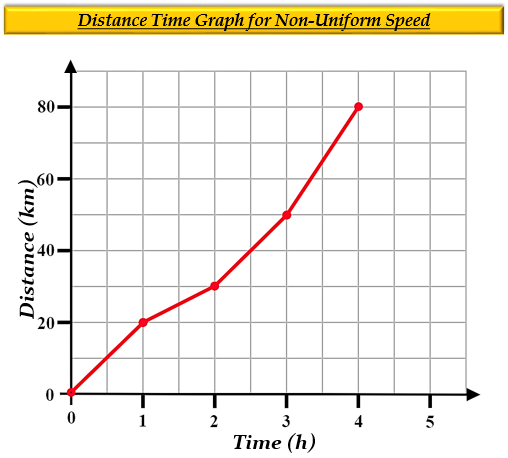# Can we calculate average speed in a distance-time-graph which is in non-uniform motion? If yes, please give an example.

Distance-time graphs - These graphs give an idea of the distance travelled by the body with respect to time. They are plotted keeping distance along y-axis (vertical line) and time along x-axis (horizontal line).

Non-Uniform Motion- A body is said to be in a non-uniform motion if it travels unequal distances in equal intervals of time. In other words, motion is said to be accelerated. Thus, a distance-time graph for non-uniform motion will be a curved line.

For example:

Suppose an object travels at the speed of 20 km/h in the first hour, 30 km/h in the second, 50 km/h in the third hour, 80 km/h in the fourth hour.

It is represented as:

 Hour Distance Travelled 0 0 1 20 2 30 3 50 4 80

In a non-uniform motion, the average speed of a body is calculated by dividing the total distance travelled by the object, with the total time taken in the journey.

Thus,

$Average\ Speed=\frac {Total\ distance\ travelled\ by\ the\ object}{Total\ time\ taken\ in\ the\ journey}$

Here,

The total distance travelled = $0+20+30+50+80=180 km$

The total time taken in the journey = $0+1+2+3+4=10 h$

Putting these values in the formula we get-

$Average\ Speed=\frac {180}{10}$

$Average\ Speed=18 km/h$Updated on: 10-Oct-2022

205 Views